# Dice and coin

A number cube is rolled and a coin is tossed. The number cube and the coin are fair. What is the probability that the number rolled is greater than 2 and the coin toss is head?

p =  0.3333

### Step-by-step explanation: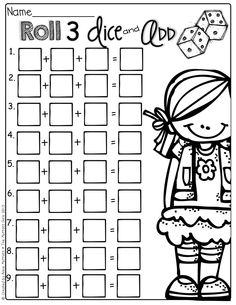Did you find an error or inaccuracy? Feel free to write us. Thank you!Tips to related online calculators
Need help to calculate sum, simplify or multiply fractions? Try our fraction calculator.
Would you like to compute count of combinations?

## Related math problems and questions:

• Dice 7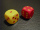A standard number cube is tossed 210 times. What is a reasonable prediction for the number of times the number cube will land on a 5?
• Two diceWe roll two dice. What is the probability that the sum of the falling numbers is greater than 3?
• The diceWhat is the probability of events that if we throw a dice is rolled less than 6?
• One dice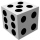Calculate the probability of one dice roll with the numbers 1, 2, 3, 4, 5, 6 on the walls. Write the results in a notebook in the shape of a fraction in the basic form: 2/3. a, The number 1 falls on the cube. b, The number 5 falls on the cube. c, An even
• DicesWe will throw two dice. What is the probability that the ratio between numbers on first and second dice will be 1:2?
• One threeWe throw two dice. What is the probability that max one three falls?
• Roll the diceWhat is the probability that if we roll the dice, a number less than 5 falls?
• Six on diceWhat is the probability that when throwing two dice will fall at least one six?
• Three dice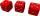What is the probability that when we roll three dice, we roll the numbers 1,2,3?
• We rollWe roll two dice A. - what is the probability that the sum of the falling numbers is at most 4 B. - is at least 10 C. - is divisible by 5?
• Perfect cubesSuppose a number is chosen at random from the set (0,1,2,3,. .. ,202). What is the probability that the number is a perfect cube?
• The diceFind the probability that a number divisible by two or three will fall when the dice are rolled.
• Sum or productWhat is the probability that two dice fall will have the sum 7 or product 12?
• Components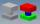In the box are 8 white, 4 blue and 2 red components. What is the probability that we pull one white, one blue and one red component without returning?
• Coin and die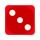Flip a coin and then roll a six-sided die. How many possible combinations are there?
• DiceWe throw five times the dice. What is the probability that six fits exactly twice?
• Fall sum or sameFind the probability that if you roll two dice, it will fall the sum of 10, or the same number will fall on both dice.